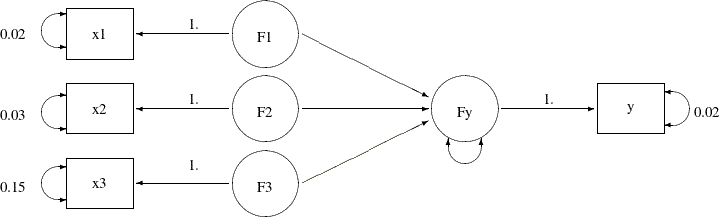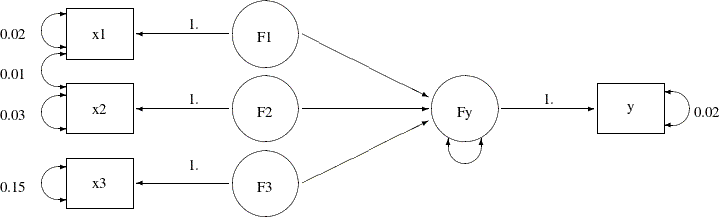### Example 27.10 Measurement Error Models with Multiple Predictors

In Example 27.8 and Example 27.9, you fit various measurement error models with only one predictor. This example illustrates the case in which you have more than one predictor, all measured with errors. The measurement errors might also be correlated.

The data from 37 observations are summarized in a covariance matrix as shown in the following SAS DATA step:

```data multiple(type=cov);
input _type_ \$ 1-4  _name_ \$ 6-8 @10 y x1 x2 x3;
datalines;
mean     0.93   1.33   1.34   4.11
cov  y   1.31    .      .      .
cov  x1  1.24   1.42    .      .
cov  x2  0.21   0.18   1.15    .
cov  x3  3.91   4.21   0.58  14.11
;
```

In this data set, four variables are measured. Variables `x1``x3` are predictors of `y`. Instead of the raw data, you can input the sample covariance matrix in the form of a SAS data set for PROC CALIS to analyze.

You assume all of these variables in the data set are measured with errors. From prior studies, you establish the knowledge about the measurement errors of these variables. You create the true score counterparts for each of these variables in the same manner as you do in Example 27.8 and Example 27.9. The following path diagram represents your measurement error model for the data:

Output 27.10.1:In the path diagram, variables `F1``F3` and `Fy` are latent variables that represent the true score for the measured indicators `x1``x3` and `y`, respectively. All paths from the true scores to the corresponding measured indicators are labeled with the fixed constant 1, as required by the measurement model. Each measured indicator is attached with a double-headed arrow that indicates the error variance. Because you have knowledge about these measurement error variances, you put fixed constant values adjacent to these double-headed arrows. For example, the measurement error variance of `y` is 0.02 and the measurement error variance of `x3` is 0.15. The path diagram also indicates that the paths from `F1``F3` to `Fy` and the error variance for `Fy` are free parameters to estimate in the model.

Notice that for brevity the variances and covariances among the three exogenous true score variables `F1``F3` are not represented in the path diagram. These six variance and covariance parameters could have been represented by double-headed arrows in the path diagram. However, because PROC CALIS always assumes the exogenous variances and covariances as default model parameters, this information is not represented to reduce clutter in the path diagram.

You can transcribe the path diagram easily to the following PATH model specification:

```proc calis data=multiple nobs=37;
path
Fy  <===   F1 F2 F3,
F1  ===>   x1   = 1.,
F2  ===>   x2   = 1.,
F3  ===>   x3   = 1.,
Fy  ===>   y    = 1.;
pvar
x1 x2 x3 y = .02 .03 .15 .02,
Fy;
run;
```

In the first entry of the PATH statement, you specify that `F1``F3` predicts `Fy`. In the next four path entries you specify the measurement model for the true scores and how they are related to the observed variables. In the PVAR statement, you specify all the known measurement error variances for the observed variables. They are all fixed constants in the model. In the last entry in the PVAR statement, you specify the error variance of `Fy` as a free (unnamed) parameter. You could have omitted this entry because error variances for all endogenous variables in the PATH model are free parameters by default. Setting these default parameters explicitly as free parameters would not affect model fitting.

Output 27.10.2 shows the parameter estimates of the model. The path coefficient or effect from `F2` to `Fy` is not significant, while the other two path coefficients are at least marginally significant.

Output 27.10.2: Parameter Estimates of the Measurement Model with Multiple Predictors

PATH List
Path Parameter Estimate Standard
Error
t Value
Fy <=== F1 _Parm1 0.46507 0.22682 2.05035
Fy <=== F2 _Parm2 0.04123 0.07069 0.58323
Fy <=== F3 _Parm3 0.13812 0.07175 1.92490
F1 ===> x1   1.00000
F2 ===> x2   1.00000
F3 ===> x3   1.00000
Fy ===> y   1.00000

Variance Parameters
Variance
Type
Variable Parameter Estimate Standard
Error
t Value
Error x1   0.02000
x2   0.03000
x3   0.15000
y   0.02000
Fy _Parm4 0.16461 0.04522 3.64028
Exogenous F1 _Add1 1.40000 0.33470 4.18289

Covariances Among Exogenous Variables
Var1 Var2 Parameter Estimate Standard
Error
t Value
F2 F1 _Add4 0.18000 0.21508 0.83688
F3 F1 _Add5 4.21000 1.02416 4.11070
F3 F2 _Add6 0.58000 0.67829 0.85509

The second table of Output 27.10.2 shows the variance estimates. As specified in the model, all measurement error variances for the observed variables are fixed constants. The error variance of `Fy` is 0.1646 (standard error =0.0452). Although you do not specify them in the PATH model specification, variances of `F1``F3` are free parameters in the model. The second table of Output 27.10.2 shows their estimates. The last table of Output 27.10.4 shows the covariances among the latent true scores. Only the covariance between `F3` and `F1` is significant.

PROC CALIS not only can handle measurement error variance with multiple true score predictors, but it also can handle correlated errors. Suppose that the measurement errors for variables `x1` and `x2` are correlated. From prior studies, you determine that their covariance is 0.01. The path diagram with this new piece of information added is shown in the following:

Output 27.10.3:In the path diagram, the double-headed arrow that connects `x1` and `x2` represents the covariance between the error terms for the two variables. The value attached to this double-headed arrow is 0.01, which represents a fixed constant in the model. The PATH model specification is similar to the preceding specification, with one more entry added in the PCOV statement, as shown in the following statements:

```proc calis data=multiple nobs=37;
path
Fy  <===   F1 F2 F3,
F1  ===>   x1   = 1.,
F2  ===>   x2   = 1.,
F3  ===>   x3   = 1.,
Fy  ===>   y    = 1.;
pvar
x1 x2 x3 y = .02 .03 .15 .02,
Fy;
pcov
x1 x2 = 0.01;
run;
```

Except for the PCOV statement specification, everything else is the same as in the preceding specification. In the PCOV statement, you can specify covariance or error covariances between exogenous or endogenous variables. In the current model, because both `x1` and `x2` are endogenous in the model, the specification is for their error covariance, which is fixed at 0.01 as required.

Output 27.10.4 shows the parameter estimates of the measurement model with correlated errors. The estimates do not change much from the preceding analysis in which correlated errors is not assumed. Perhaps the correlation between the errors in the current model is so small that it is ignorable. The last table in Output 27.10.4 shows the covariance estimates among errors. This table is unique to the current model. It shows that the measurement errors for `x1` and `x2` have a covariance of 0.01, which is treated as a fixed constant in the current model.

Output 27.10.4: Parameter Estimates of the Measurement Model with Multiple Predictors: Correlated Errors

PATH List
Path Parameter Estimate Standard
Error
t Value
Fy <=== F1 _Parm1 0.46839 0.22695 2.06386
Fy <=== F2 _Parm2 0.04549 0.07074 0.64306
Fy <=== F3 _Parm3 0.13694 0.07194 1.90351
F1 ===> x1   1.00000
F2 ===> x2   1.00000
F3 ===> x3   1.00000
Fy ===> y   1.00000

Variance Parameters
Variance
Type
Variable Parameter Estimate Standard
Error
t Value
Error x1   0.02000
x2   0.03000
x3   0.15000
y   0.02000
Fy _Parm4 0.16421 0.04523 3.63046
Exogenous F1 _Add1 1.40000 0.33470 4.18289

Covariances Among Exogenous Variables
Var1 Var2 Parameter Estimate Standard
Error
t Value
F2 F1 _Add4 0.17000 0.21508 0.79039
F3 F1 _Add5 4.21000 1.02416 4.11070
F3 F2 _Add6 0.58000 0.67829 0.85509

Covariances Among Errors
Error of Error of Estimate Standard
Error
t Value
x1 x2 0.01000

This example shows how you can use PROC CALIS to fit measurement error models with multiple true score predictors. You can also fit models with correlated errors. The model specification tool is the PATH modeling language, which ties closely to the path diagram representations.

However, some researchers might prefer to use linear equations to represent the measurement error models. PROC CALIS provides the LINEQS modeling language for specifying the measurement error models, or mean and covariance structure models in general. Example 27.11 illustrates the LINEQS model specification of the measurement error models.